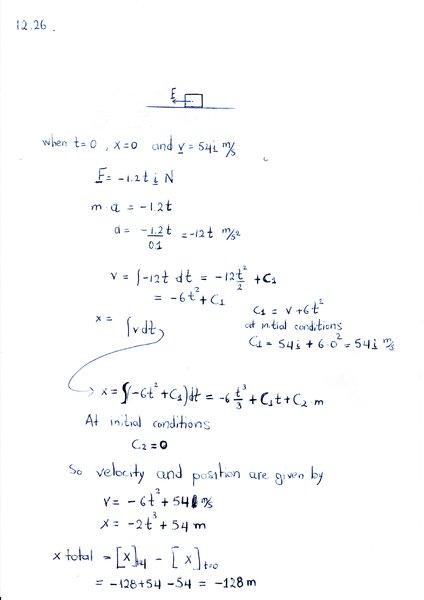# Pytels Dynamics 12.26: Block moves along the x-axis

## Homework Statement

A 0.1-kg block moves along the x-axis. The resultant of all forces acting on the block is F = -1.2tiN, where t
is in seconds. When t=0, x=0 and v=54i m/s.
Determine the distance traveled by the particle during the time interval t=0 to t=4s.

## The Attempt at a Solution

See the attached file.

Also, when do I use vector notation in the equations? Is it my choice whether to use it or not?
Next problem is with better pen.

#### Attachments

•Pytel_Dynamics025.jpg
17.5 KB · Views: 433

ehild
Homework Helper

## Homework Statement

A 0.1-kg block moves along the x-axis. The resultant of all forces acting on the block is F = -1.2tiN, where t
is in seconds. When t=0, x=0 and v=54i m/s.
Determine the distance traveled by the particle during the time interval t=0 to t=4s.

## The Attempt at a Solution

See the attached file.

Also, when do I use vector notation in the equations? Is it my choice whether to use it or not?
Next problem is with better pen.The x(t) function is wrong.
The problem asks the distance traveled. It is not the same as the displacement, x(final)-x(initial).

The book gives a result of 128m.
Basicallly I am confused on how to calculate this. Do I calculate the position at every second (from 0 to 4 seconds) and then...?
Also x(t) should be ##x(t)=-2t^3+54t##
By looking the graph of the function when v=0 then t=3 and could be easily calculated.
At t=3s then x=108m. At t=4s then x=88m.
So the block had travelled from t=0s to t=3s to the right for 108m and then from t=3s to t=4s to the left for 20m. So distance travelled
from t=0s to t=4s is 128m.
How do I calculate this by using a formula?

ehild
Homework Helper
The book gives a result of 128m.
Basicallly I am confused on how to calculate this. Do I calculate the position at every second (from 0 to 4 seconds) and then...?
Also x(t) should be ##x(t)=-2t^3+54t##
By looking the graph of the function when v=0 then t=3 and could be easily calculated.
At t=3s then x=108m. At t=4s then x=88m.
So the block had travelled from t=0s to t=3s to the right for 108m and then from t=3s to t=4s to the left for 20m. So distance travelled
from t=0s to t=4s is 128m.
How do I calculate this by using a formula?
The distance traveled is the integral of the speed with respect to time. The speed is magnitude of the velocity ##v=|\vec v|##. The velocity changes sign at t = 3 s, so ##d=\int _0^3(-6t^2+54)dt+\int _3^4(6t^2-54)dt##

•Alexanddros81
Yes. I've done the math and works fine. 108 from forst integral and 20 from second.